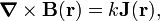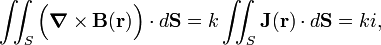# Ampere's law

In physics, or more in particular in electrodynamics, Ampère's law relates the strength of a magnetic field to the electric current that causes it. The law was first formulated by André-Marie Ampère around 1825. Later (1864) it was augmented by James Clerk Maxwell, who added displacement current to it. This extended form is one of the four Maxwell's equations that form the axiomatic basis of electrodynamics.

## Formulation

We consider a closed curve C around an electric current i. Other than the current i there is no matter present; this is the microscopic (or vacuum) formulation of Ampère's law. Mathematically the law reads$\oint_C \mathbf{B}\cdot d\mathbf{s} = k i,$

where k = μ0 for SI units and k = 4π/c for Gaussian units. Here μ0 is the magnetic constant (also known as vacuum permeability), and c is the speed of light. The vector field B is known as the magnetic induction. The direction of integration along C matches i in the sense of a right-handed screw. Drive such a screw in the direction of positive current i (which is from + to − voltage) then the direction of rotation of the screw is the direction of integration along C. Equivalently: curve your right hand around C and integrate from wrist to fingertips, then i runs in the direction of your stretched thumb.

## Relation with Maxwell's equation

Ampère's law follows from the following Maxwell equation with zero displacement current:$\boldsymbol{\nabla}\times \mathbf{B}(\mathbf{r}) = k \mathbf{J}(\mathbf{r}),$

where$\boldsymbol{\nabla}\times \mathbf{B}$ is the curl of the vector field B(r) and J(r) is the current density (amount of charge passing through a unit surface in unit time, i.e., electric current per unit surface). Further k = μ0 for SI units and k = 4π/c for Gaussian units. Integrate both sides over a surface S and note that the infinitesimal element dS is by definition a vector with length equal to the surface of the infinitesimal element and direction normal (perpendicular) to the element. The inner product$\mathbf{J}\cdot d\mathbf{S}$ is the current di through dS and the surface integral is the total current i through S. Hence,$\iint_S \Big(\boldsymbol{\nabla}\times \mathbf{B}(\mathbf{r})\Big)\cdot d\mathbf{S} = k \iint_S \mathbf{J}(\mathbf{r})\cdot d\mathbf{S} = k i,$

Applying to the left-hand side Stokes' theorem that reads for any vector field A,$\iint_{S} \Big(\boldsymbol{\nabla}\times \mathbf{A}(\mathbf{r})\Big) \cdot d\mathbf{S} = \oint_C \mathbf{A}\cdot d\mathbf{s},$

where S is the surface bordered by the closed curve C, we find$\oint_C \mathbf{B}\cdot d\mathbf{s} = k i,$

which is indeed Ampère's law.